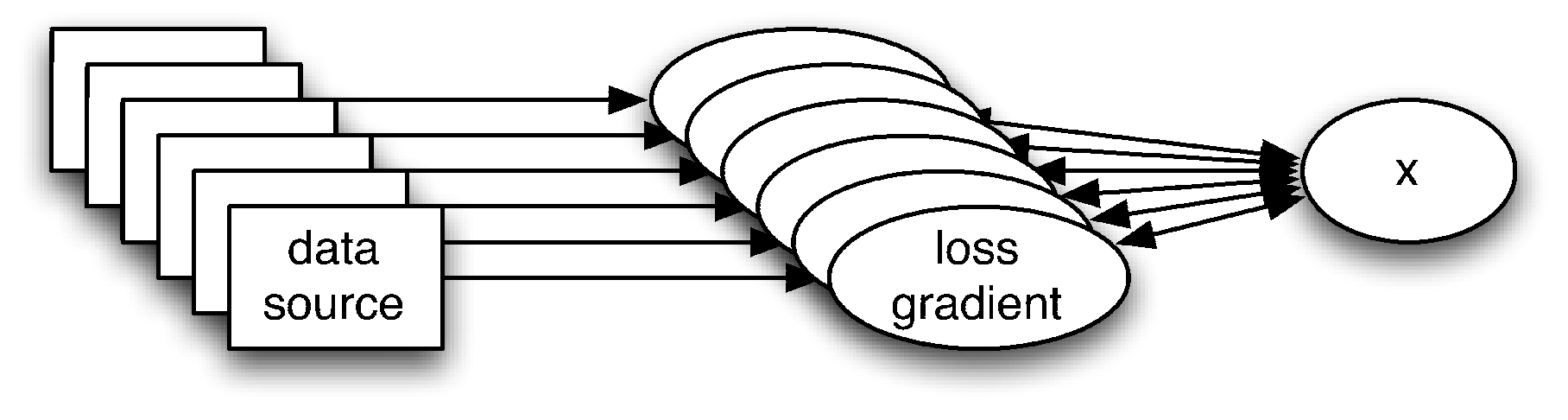distributed learning
optimization
Published

April 1, 2011Here’s the problem: you’ve optimized your stochastic gradient descent library but the code is still not fast enough. When streaming data off a disk/network you cannot exceed 100 MB/s, so processing a 1TB of data will take about 3-5 hours on a single machine (note: these were 2009 numbers - by now SSDs regularly clock in at over 8GB/s, about two orders of magnitude faster, but the datasets have grown accordingly). And classical stochastic gradient descent is sequential even in the context of multicore machines. Before looking at ways to speed up the algorithm let’s look at the basic SGD template:

$w \leftarrow w - \eta_t \lambda \partial_w \Omega[w] - \eta_t \partial_w f_t(w)$

Here $$f(w)$$ is the loss function and $$\Omega[w]$$ is the regularizer. This procedure is entirely sequential, so how to accelerate it?

### Multicore

One option is to execute the above loop in each core independently while working with a common shared weight vector $$w$$. This means that if we update $$w$$ in a round-robin fashion we will have a delay of $$k-1$$ when using $$k$$ cores. The delay is due to the time it takes between seeing an instance of $$f$$ and when we can actually apply the update to $$w$$. The beauty of this approach is that one can prove convergence (thanks to Marty Zinkevich and John Langford) without losing anything in the rates relative to the single core setting. The intuition behind it is that as we converge optimization becomes more and more an averaging procedure and in this case a small delay does not hurt at all.

### Multiple Machines

The Achilles heel of the above algorithm is that it requires extremely low latency between the individual processing nodes. While this is OK on a computer or on a GPU, it will fail miserably on a network of computers since we may have many miliseconds latency between the nodes. This suggests an even simpler algorithm for further parallelization:

1. Overpartition the data into $$k$$ blocks for $$k$$ clusters, i.e. for each cluster simply randomly draw a fraction $$c = O(m/k)$$ of the entire dataset.
2. Now perform stochastic gradient descent on each machine separately with constant learning rate.
3. Average the solutions between different machines.

Surprisingly enough this method can be shown to converge and give optimal speedup. The aforementioned paper with Marty Zinkevich, Markus Weimer and Lihong Li has the proof. A related paper by Niu, Recht, Re and Wright, 2011 describing Hogwild uses fully asynchronous updates.